# Comparison of Auto White Balance Algorithms

Our eyes are very good at judging what is white under different lighting conditions. Digital cameras, however, without some kind of adjustment, can easily capture unrealistic images with a strong color cast. Automatic white balance (AWB) algorithms try to correct for the ambient light with minimum input from the user, so that the resulting image looks like what our eyes would see. But which is the best AWB algorithm to use? In this example, we explain the process behind auto white balance and show how to compare and select the best algorithm.

Automatic white balancing is done in two steps:

• Step 1: Estimate the scene illumination.

• Step 2: Correct the color balance of the image.

The hardest step, and the one we will focus on optimizing, is Step 1 -- the estimation of the ambient light. Once the ambient light is known, then correcting the colors in the image (Step 2) is an easy and fixed process.

In the following, we judge the quality of three algorithms for illuminant estimation by comparing them to the ground truth scene illumination:

• White Patch Retinex 

• Gray World 

• Cheng's Principal Component Analysis (PCA) method 

### Read 16-bit Image of Linear RGB Values

Auto white balance algorithms are usually applied on the raw image data, before the image is compressed and saved to the memory card. `foosballraw.tiff` is an image file that contains such raw sensor data after correcting the black level and scaling the intensities to 16 bits per pixel. This image is free of the white balancing done by the camera, as well as demosaicking, denoising, chromatic aberration compensation, tone adjustments, gamma correction and other processing done before the image is saved to the memory card.

`A = imread('foosballraw.tiff');`

The image `A` contains linear RGB values. Most illuminant estimation algorithms assume a linear relationship between the response of the imaging sensor and pixel intensities. However, because of the nonlinear nature of display devices, image files meant to be displayed such as JPEG files incorporate a gamma correction. Without gamma correction images would look very dim on a computer screen. If you are working with gamma-corrected images, make sure to linearize them with the `rgb2lin` function. This is not the case with `foosballraw.tiff`, so skip this step.

### Interpolate to Recover Missing Color Information

Digital cameras use a color filter array superimposed on the imaging sensor to simulate color vision, so that each pixel is sensitive to either red, green or blue. To recover the missing color information at every pixel, you must interpolate. The Bayer pattern used by the camera with which the photo was captured (Canon EOS 30D) is RGGB.

`A = demosaic(A,'rggb');`

### Gamma-Correct the Image for Display

If you try to display the linear image as-is, it will appear very dim, because of the non-linear characteristic of display devices. Therefore, for display purposes, gamma-correct the image to use the sRGB color space.

`A_sRGB = lin2rgb(A);`

Display the original, minimally processed image before and after gamma correction

```warning('off','images:initSize:adjustingMag') montage({A,A_sRGB}) title('Original, minimally processed image before and after gamma correction')```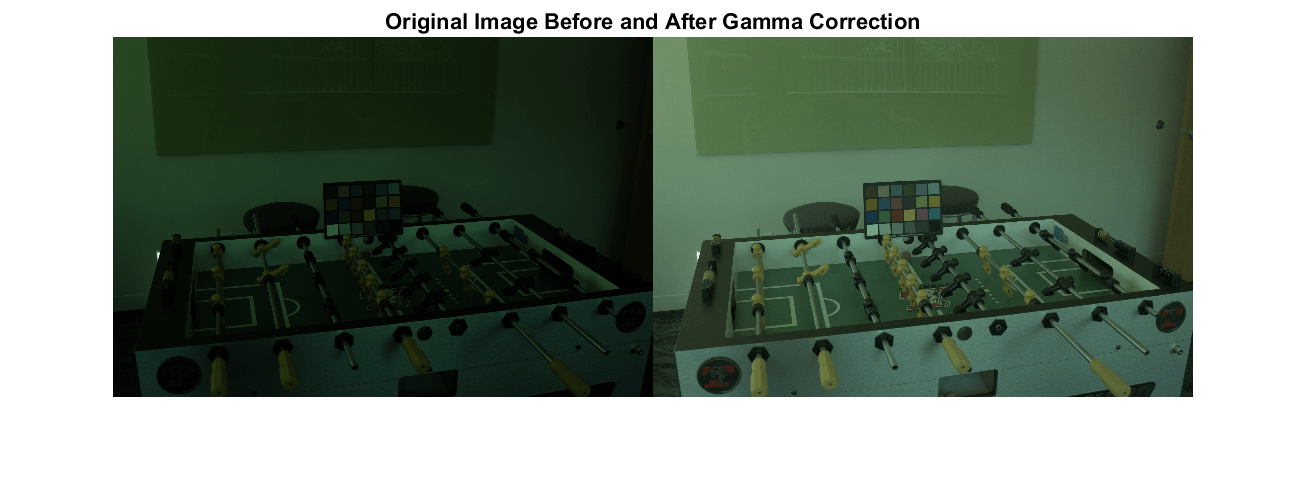### Locate the ColorChecker Chart

A ColorChecker Chart has been included in the scene. This chart is made of 24 neutral and color patches with known spectral reflectances. We will use the 6 neutral (achromatic) patches on the bottom row to estimate the ground truth illumination in the scene, against which the algorithms will be compared. However, when testing the algorithms, the chart must be excluded to prevent the algorithms to unfairly take advantage of it – there is no ColorChecker Chart in real-life situations.

Specify the location of the ColorChecker Chart. By default, the example provides the cooridinates of a bounding quadrilateral. If you want to select the polygon coordinates interactively, change the value of `select_polygon` to `true`.

```select_polygon = false; if select_polygon % Use roipoly to create a mask from a polygon drawn manually. % Click to add vertices, then right-click and select "Create Mask" to return. imshow(A_sRGB) title('Draw a polygon around the chart') mask_chart = roipoly; else % Use the provided coordinates of the bounding rectangle. c = [930 1280 1316 953]; r = [1877 1890 1382 1370]; mask_chart = roipoly(A_sRGB,r,c); end```

Dilate the mask slightly to ensure we do not include any pixels belonging to the chart.

`mask_chart = imdilate(mask_chart,ones(7));`

### Measure the Ground Truth Illumination Using the ColorChecker Chart

For the purpose of white balance we will only use the 6 neutral patches on the bottom row of the chart. These neutral patches reflect light equally across the visible spectrum. They reflect the illumination of the scene. The ground truth illuminant is computed as the average color of the neutral patches, excluding under- and over-exposed pixels.

Specify the center of each neutral patch. By default, the example provides an estimate of the center coordinate of each patch. If you want to select the ROI centers interactively, change the value of `estimate_roi_centers` to `true`.

```estimate_roi_centers = false; if estimate_roi_centers % Zoom in and click the center of each of the 6 neutral patches. xlim([1350 1930]) ylim([900 1350]) title('Click the center of each of the 6 neutral patches') [x,y] = ginput(6); else % Use the provided estimate of ROI center coordinates. x = [1424 1514 1598 1676 1757 1835]; y = [1268 1250 1247 1250 1235 1229]; end```

We derive a square area from the coordinates of the center of each patch by considering the side of a square to be 80% of the distance separating the centers of two consecutive patches.

```x = round(x); y = round(y); r = mean(diff(x)) / 2 * 0.80; r = floor(r);```

Create a binary mask covering the neutral patches.

```mask = false(size(A,1), size(A,2)); for k = 1:6 mask(y(k)-r:y(k)+r,x(k)-r:x(k)+r) = true; end```

Erode the mask to avoid including pixels outside of the patches or on the border, which are prone to chromatic aberration, whose colors can skew the measurement of the ground truth.

`mask_eroded = imerode(mask, strel('disk',5));`

Identify saturated RGB values in the input image. These values should be excluded from the computation of the ground truth as they will skew the measurement as well.

```mask_clipped = (A == intmax(class(A))) | (A == intmin(class(A))); mask_clipped = mask_clipped(:,:,1) | mask_clipped(:,:,2) | mask_clipped(:,:,3);```

Exclude these clipped pixels from the mask corresponding to the neutral patches.

`mask_patches = mask_eroded & ~mask_clipped;`

Visualize the selected pixels. The highlighted pixels should all be within the neutral patches. If not, try clicking the centers of the patches again and repeat the steps above.

```A_patches = imoverlay(A_sRGB,mask_patches); imshow(A_patches) title('The selected pixels are highlighted in yellow')```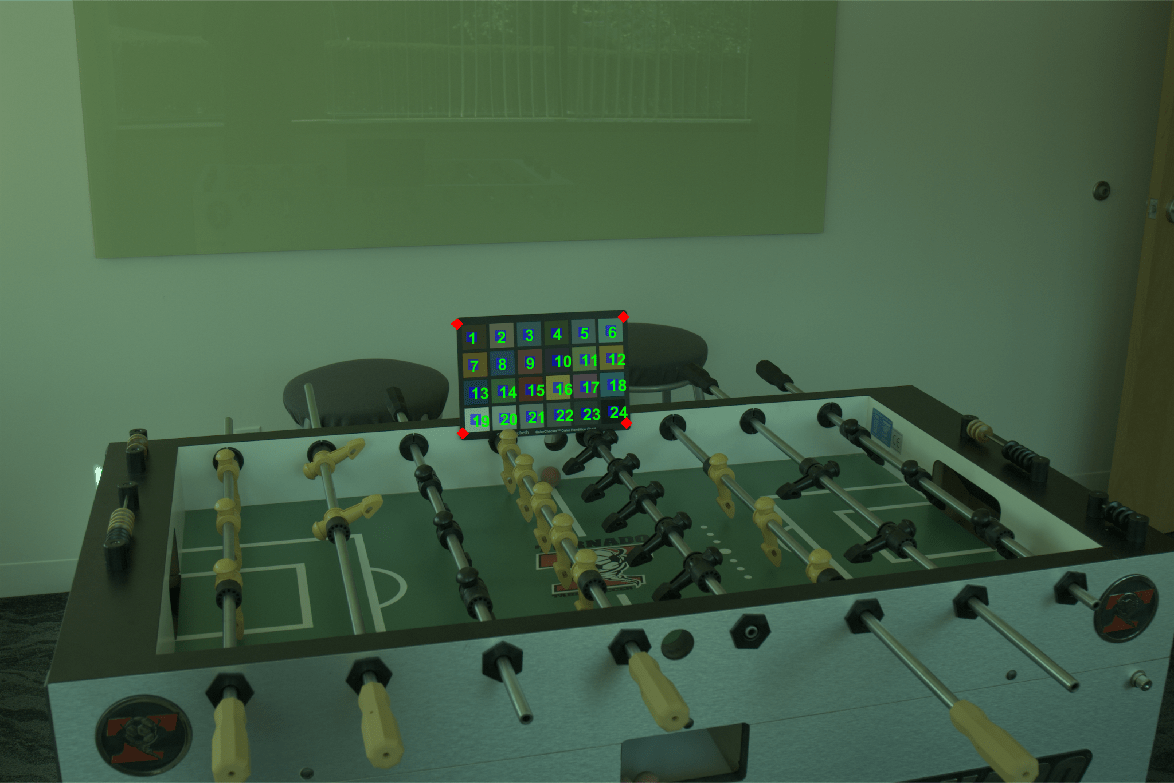Obtain the red, green and red values for the neutral patches.

```patches_R = A(:,:,1); patches_G = A(:,:,2); patches_B = A(:,:,3); patches_R = patches_R(mask_patches); patches_G = patches_G(mask_patches); patches_B = patches_B(mask_patches);```

For simplicity and because most illuminant estimation algorithms work in floating point, we convert the RGB values of the patches to double and scale the values to be in [0 1] before computing the mean.

```patches_R = im2double(patches_R); patches_G = im2double(patches_G); patches_B = im2double(patches_B);```

Compute the ground truth RGB illuminant as the mean RGB value across the neutral patches.

`illuminant_groundtruth = [mean(patches_R) mean(patches_G) mean(patches_B)];`

### Angular Error

To compare an estimated illuminant against the ground truth, compute the angular error between the two colors. To better understand the concept of angular error, consider the following visualization of an arbitrary illuminant and the ground truth we just measured. Each illuminant represents a vector in RGB space.

```illuminant = [0.066 0.1262 0.0691]; plot3([0 1],[0 1],[0,1],'LineStyle',':','Color','k') hold on plot3(... [0 illuminant_groundtruth(1)/norm(illuminant_groundtruth)], ... % Red [0 illuminant_groundtruth(2)/norm(illuminant_groundtruth)], ... % Green [0 illuminant_groundtruth(3)/norm(illuminant_groundtruth)], ... % Blue 'Marker','.', 'MarkerSize',10) hold on plot3( ... [0 illuminant(1)/norm(illuminant)], ... % Red [0 illuminant(2)/norm(illuminant)], ... % Green [0 illuminant(3)/norm(illuminant)], ... % Blue 'Marker','.', 'MarkerSize',10) xlabel('R') ylabel('G') zlabel('B') title('Illuminants in RGB space') xlim([0 1]) ylim([0 1]) zlim([0 1]) view(28, 36) legend('achromatic line', 'ground truth illuminant', 'estimated illuminant') grid on axis equal```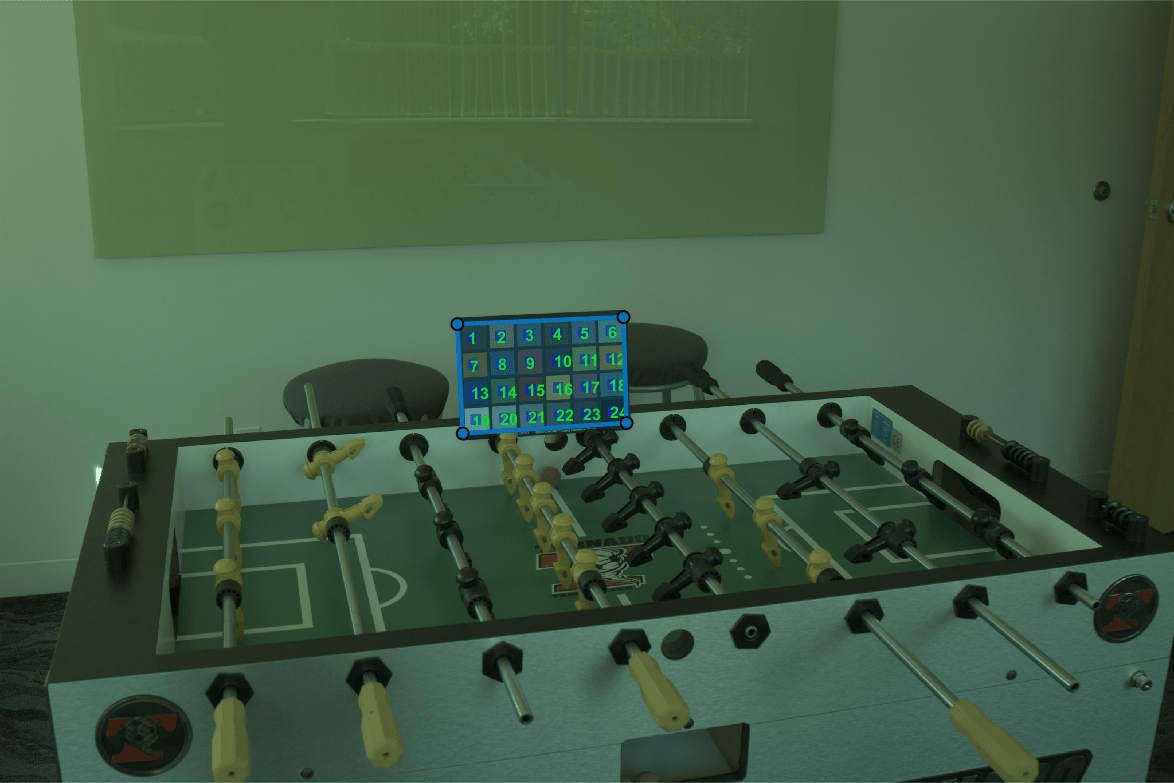The exact value of the estimated illuminant does not matter as much as its direction, since the direction of the illuminant is what is used to white balance an image. Ideally, the estimated illuminant should be aligned with the ground truth illuminant. The angular error between the estimated illuminant and the ground truth is the angle (in degrees) formed by the two vectors. The smaller the angular error, the better the estimation is. We will evaluate the quality of an estimation according to the angular error against the ground truth.

### White Patch Retinex

The White Patch Retinex method  for illuminant estimation assumes that the scene contains a bright patch. This patch reflects the maximum light possible for each color band, which is the color of the illumination of the scene.

The `illumwhite` function implements the White Patch Retinex method while also providing the ability to exclude part of the brightest pixels from the computation, in order to avoid taking over-exposed pixels into consideration.

First, estimate the illumination of the scene using all the pixels in the image, minus the ColorChecker Chart. This is done by specifying the top percentile to exclude to be 0. The `'Mask'` name-value pair is used to specify which pixels to use for the computation, which in our case are pixels not belonging to the ColorChecker Chart.

`illuminant_wp1 = illumwhite(A, 0, 'Mask', ~mask_chart);`

Compute the angular error for the illuminant estimated with White Patch Retinex.

```err_wp1 = colorangle(illuminant_wp1, illuminant_groundtruth); disp(['Angular error for White Patch with percentile=0: ' num2str(err_wp1)])```
```Angular error for White Patch with percentile=0: 16.5163 ```

To white balance the image (Step 2 of AWB) using this estimated illuminant, use the `chromadapt` function, which by default scales the colors in the Bradford cone response model. Make sure to specify that we are working with linear RGB color values.

`B_wp1 = chromadapt(A, illuminant_wp1, 'ColorSpace', 'linear-rgb');`

Display the gamma-corrected white balanced image

```B_wp1_sRGB = lin2rgb(B_wp1); figure imshow(B_wp1_sRGB) title('White balanced image using White Patch Retinex with percentile=0')```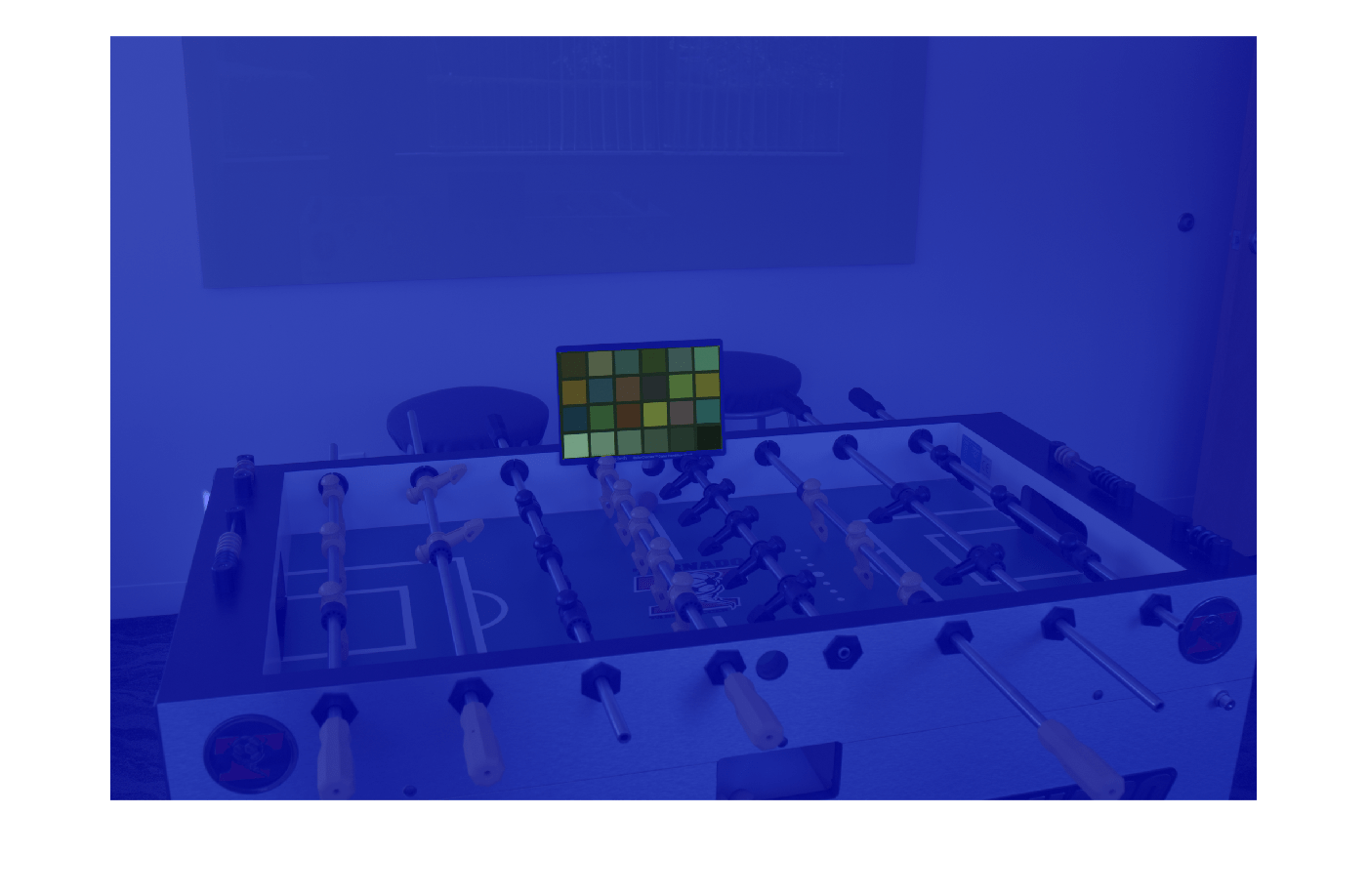Second, since selecting the maximum RGB value is sensitive to over- exposed pixels, the White Patch Retinex algorithm can be made more robust by excluding a certain percentage of the brightest pixels from the computation. This is achieved through the percentile parameter of the illumwhite function. Choose a percentile value of 1. (The default is 1.)

`illuminant_wp2 = illumwhite(A, 1, 'Mask', ~mask_chart);`

Compute the angular error for the illuminant estimated with the more robust version of White Patch Retinex.

```err_wp2 = colorangle(illuminant_wp2, illuminant_groundtruth); disp(['Angular error for White Patch with percentile=1: ' num2str(err_wp2)])```
```Angular error for White Patch with percentile=1: 5.0323 ```

Display the gamma-corrected white balanced image with the new illuminant

```B_wp2 = chromadapt(A, illuminant_wp2, 'ColorSpace', 'linear-rgb'); B_wp2_sRGB = lin2rgb(B_wp2); imshow(B_wp2_sRGB) title('White balanced image using White Patch Retinex with percentile=1')```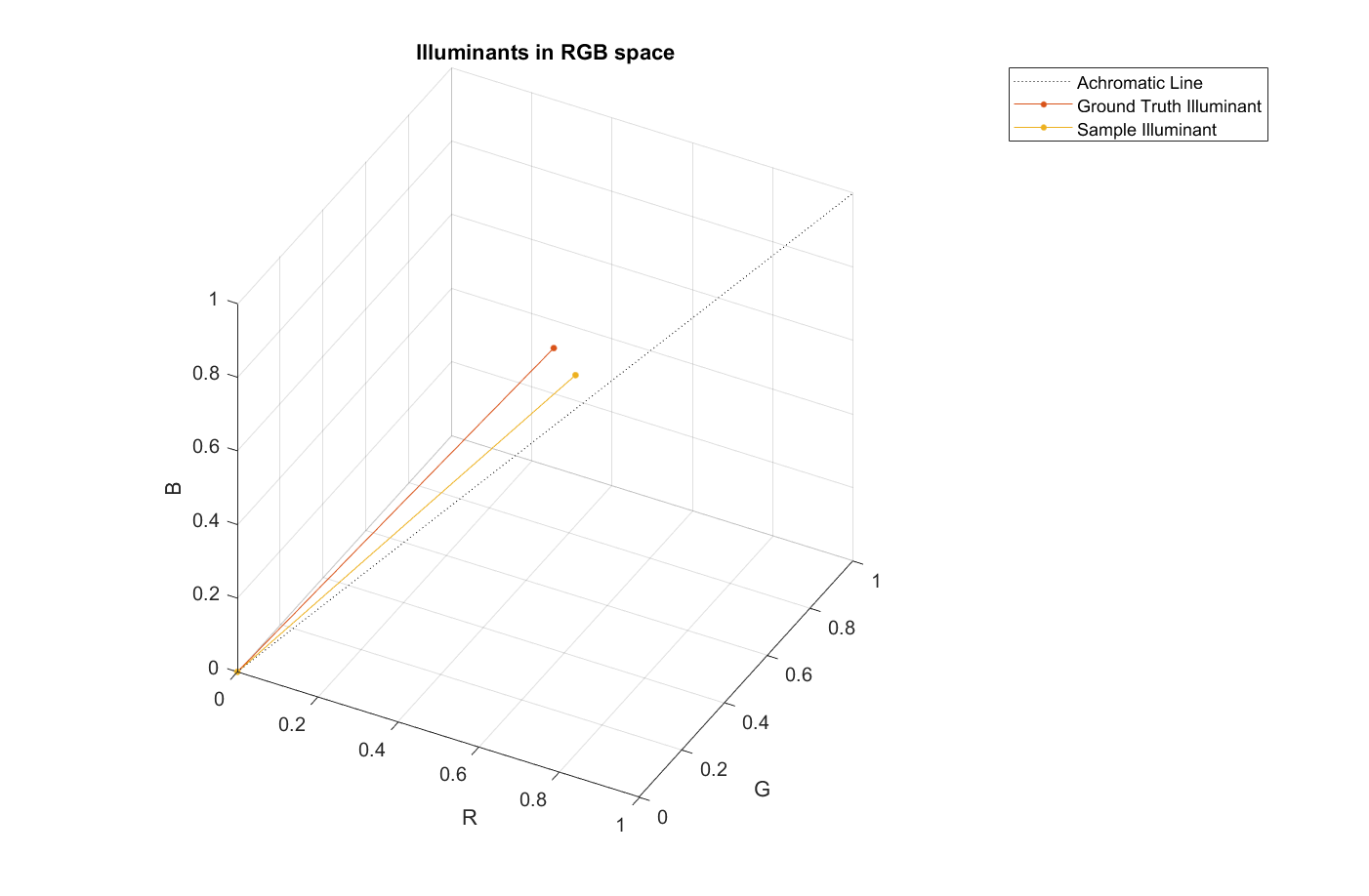### Gray World

Gray World  is most certainly the best known illuminant estimation method. It assumes that the average color of the world is gray, i.e. achromatic. Therefore, it calculates the scene illuminant as the average RGB value in the image.

The `illumgray` function implements the Gray World algorithm with one addition: It provides the ability to exclude the darkest and brightest pixels from the computation, which may skew the estimation of the illuminant, making the algorithm more robust.

First, estimate the scene illumination using all pixels of the image, excluding those corresponding to the ColorChecker Chart. The `illumgray` function provides a parameter to specify the percentiles of bottom and top values (ordered by brightness) to exclude. Here, we specify the percentiles as [0 0].

`illuminant_gw1 = illumgray(A, 0, 'Mask', ~mask_chart);`

Compare the estimated illuminant to the ground truth by computing the angular error between the two, similarly to what we did for White Patch Retinex.

```err_gw1 = colorangle(illuminant_gw1, illuminant_groundtruth); disp(['Angular error for Gray World with percentiles=[0 0]: ' num2str(err_gw1)])```
```Angular error for Gray World with percentiles=[0 0]: 5.063 ```

Apply chromatic adaptation to white balance the image with the estimated illuminant.

`B_gw1 = chromadapt(A, illuminant_gw1, 'ColorSpace', 'linear-rgb');`

Display the gamma-corrected white balanced image

```B_gw1_sRGB = lin2rgb(B_gw1); imshow(B_gw1_sRGB) title('White balanced image using Gray World with percentiles=[0 0]')```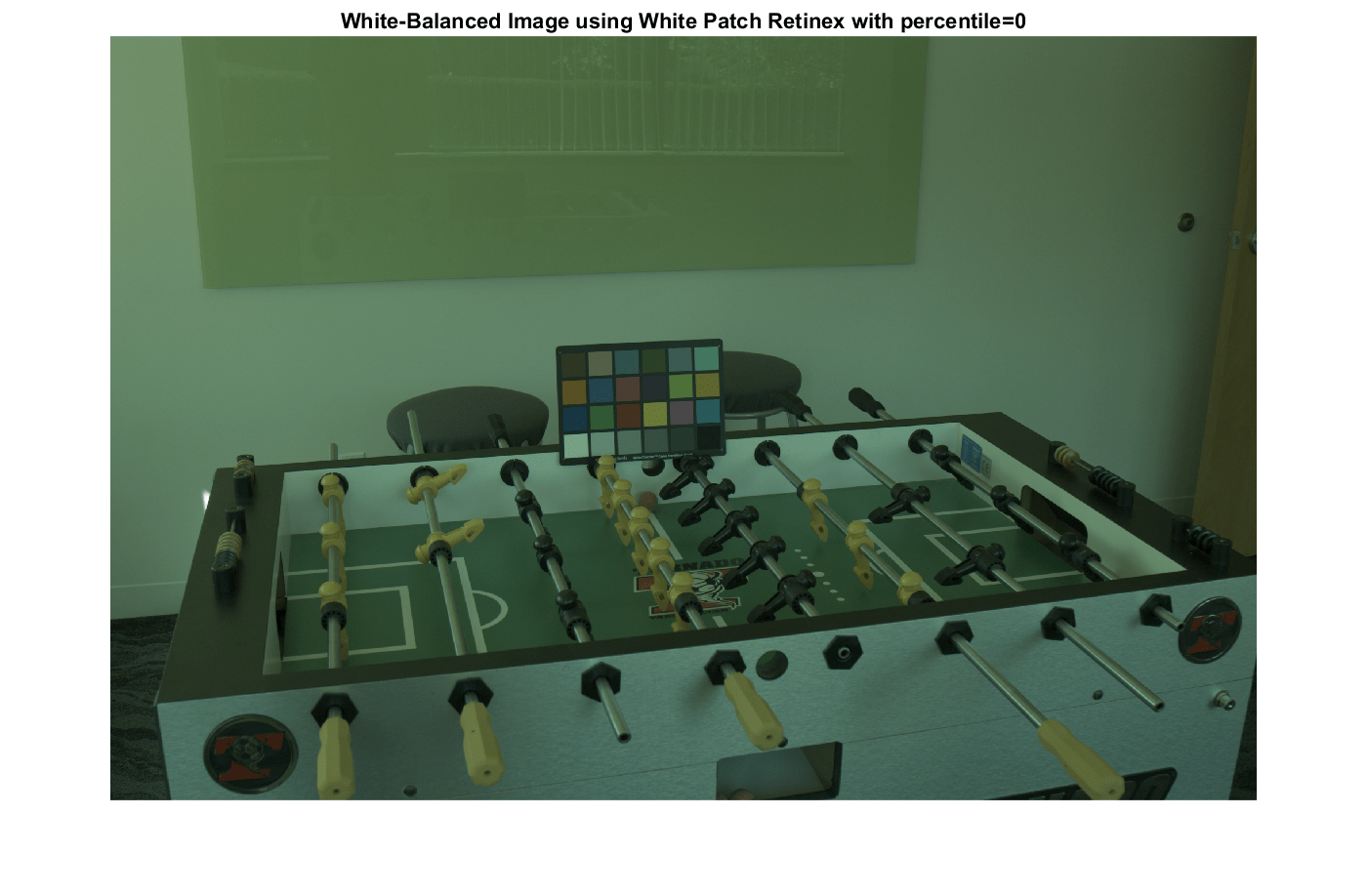Second, since under- and over-exposed pixels can negatively influence the estimation of the illuminant as the mean RGB value in the image, let's exclude the bottom and top 1% pixels. (The default value for `percentiles` is [1 1].)

`illuminant_gw2 = illumgray(A, 1, 'Mask', ~mask_chart);`

Compute the angular error for the second illuminant estimated with Gray World.

```err_gw2 = colorangle(illuminant_gw2, illuminant_groundtruth); disp(['Angular error for Gray World with percentiles=[1 1]: ' num2str(err_gw2)])```
```Angular error for Gray World with percentiles=[1 1]: 5.1314 ```

Display the gamma-corrected white balanced image with the new illuminant.

```B_gw2 = chromadapt(A, illuminant_gw2, 'ColorSpace', 'linear-rgb'); B_gw2_sRGB = lin2rgb(B_gw2); imshow(B_gw2_sRGB) title('White balanced image using Gray World with percentiles=[1 1]')```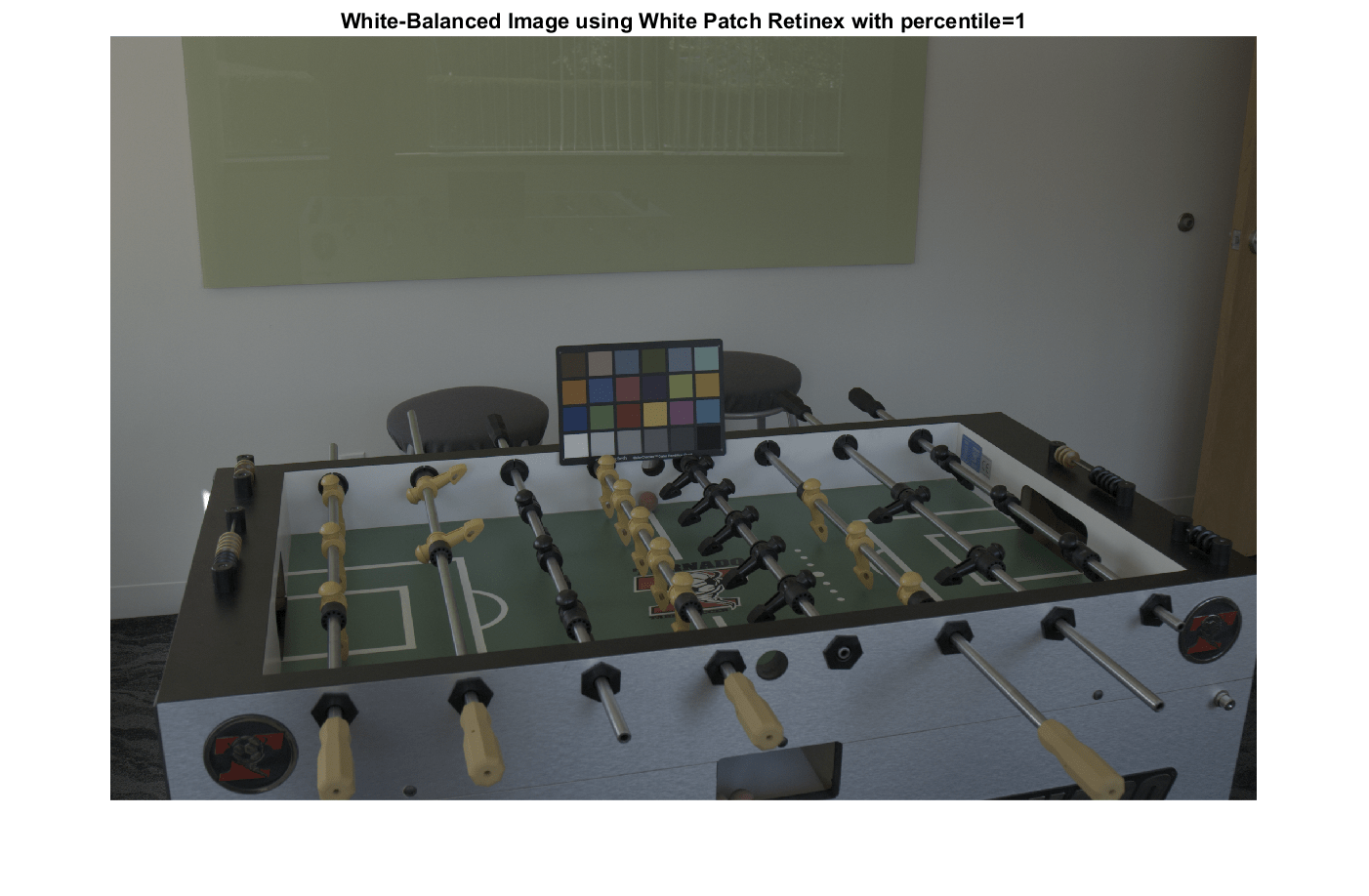### Cheng's Principal Component Analysis (PCA) method

Cheng's illuminant estimation method  draws inspiration from spatial domain methods such as Grey Edge , which assumes that the gradients of an image are achromatic. They show that Grey Edge can be improved by artificially introducing strong gradients by shuffling image blocks, and conclude that the strongest gradients follow the direction of the illuminant. Their method consists in ordering pixels according to the norm of their projection along the direction of the mean image color, and retaining the bottom and top p%. These two groups correspond to strong gradients in the image. Finally, they perform a principal component analysis (PCA) on the retained pixels and return the first component as the estimated illumination.

Cheng's method is implemented by the `illumpca` function. We use Cheng's method to estimate the illuminant in the scene using the bottom and top 5% of pixels along the direction of the mean color. (The default is 3.5.)

`illuminant_ch1 = illumpca(A, 5, 'Mask', ~mask_chart);`

Compare this estimation to the ground truth.

```err_ch1 = colorangle(illuminant_ch1, illuminant_groundtruth); disp(['Angular error for Cheng with percentage=5: ' num2str(err_ch1)])```
```Angular error for Cheng with percentage=5: 4.7595 ```

Display the gamma-corrected white balanced image

```B_ch1 = chromadapt(A, illuminant_ch1, 'ColorSpace', 'linear-rgb'); B_ch1_sRGB = lin2rgb(B_ch1); imshow(B_ch1_sRGB) title('White balanced image using Cheng with percentage=5')```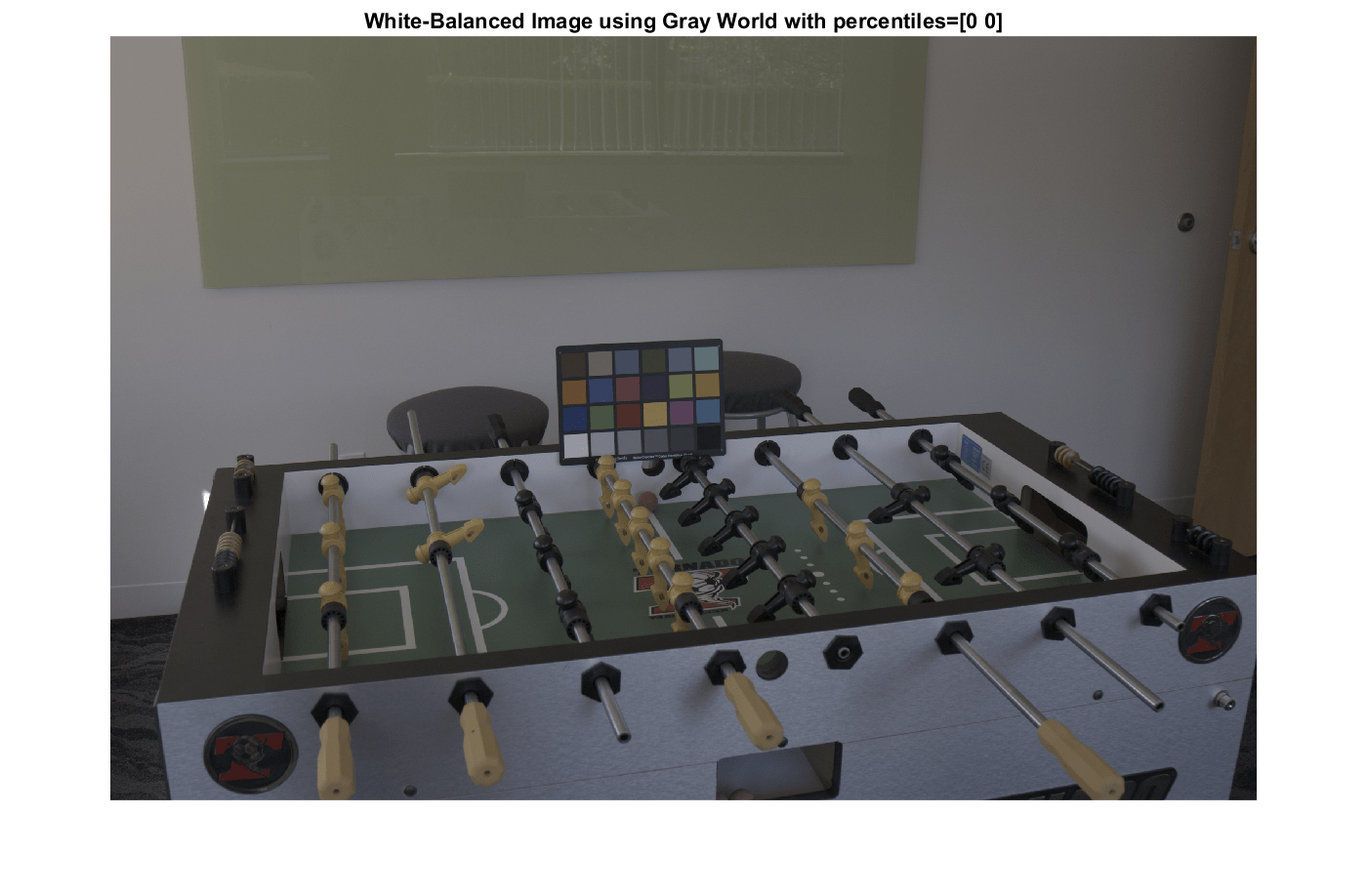Let's now use the default percentage value and see how it compares.

```illuminant_ch2 = illumpca(A, 'Mask', ~mask_chart); err_ch2 = colorangle(illuminant_ch2, illuminant_groundtruth); disp(['Angular error for Cheng with percentage=3.5: ' num2str(err_ch2)])```
```Angular error for Cheng with percentage=3.5: 5.0283 ```

Display the corrected image in sRGB.

```B_ch2 = chromadapt(A, illuminant_ch2, 'ColorSpace', 'linear-rgb'); B_ch2_sRGB = lin2rgb(B_ch2); imshow(B_ch2_sRGB) title('White balanced image using Cheng with percentile=3.5')```### Parameter Sweep

To find the best parameter to use for each method we can sweep through a range and calculate the angular error for each of them. The parameters of the three algorithms have different meanings, but the similar ranges of these parameters makes it easy to programmatically search for the best one for each algorithm.

```param_range = 0:0.25:5; err = zeros(numel(param_range),3); for k = 1:numel(param_range) % White Patch illuminant_wp = illumwhite(A, param_range(k), 'Mask', ~mask_chart); err(k,1) = colorangle(illuminant_wp, illuminant_groundtruth); % Gray World illuminant_gw = illumgray(A, param_range(k), 'Mask', ~mask_chart); err(k,2) = colorangle(illuminant_gw, illuminant_groundtruth); % Cheng if (param_range(k) ~= 0) illuminant_ch = illumpca(A, param_range(k), 'Mask', ~mask_chart); err(k,3) = colorangle(illuminant_ch, illuminant_groundtruth); else % Cheng's algorithm is undefined for percentage=0. err(k,3) = NaN; end end```

Create a visualization of the angular error as heat map.

```err_normalized = mat2gray(log(err)); block_size_x = 120; block_size_y = 50; err_image = ones(size(err,1) * block_size_y, size(err,2) * block_size_x); for i = 0:size(err,1)-1 for j = 0:size(err,2)-1 err_image(block_size_y*i+1:block_size_y*(i+1), block_size_x*j+1:block_size_x*(j+1)) = err_normalized(i+1,j+1); end end```

Display the heat map of the angular error. Light blue colors indicate a low angular error (good), while red colors indicate a high angular error (bad).

```old_pref = iptgetpref('ImshowAxesVisible'); iptsetpref('ImshowAxesVisible','on') imshow(err_image, 'Colormap', cool) iptsetpref('ImshowAxesVisible',old_pref) for i = 0:size(err,1)-1 for j = 0:size(err,2)-1 y = block_size_y*i + 1 + block_size_y/2; x = block_size_x*j + 1 + block_size_x/3; text(x,y,sprintf('%1.2f',err(i+1,j+1))) end end box off title('Angular Error') ylabel('Parameter') yticks(linspace(block_size_y/2, size(err_image,1) - block_size_y/2, numel(param_range))) yticklabels(arrayfun(@(x) {num2str(x)}, param_range)) xticks(block_size_x/2 + [0 block_size_x 2*block_size_x]) xticklabels({'White Patch','Gray World','Cheng'})```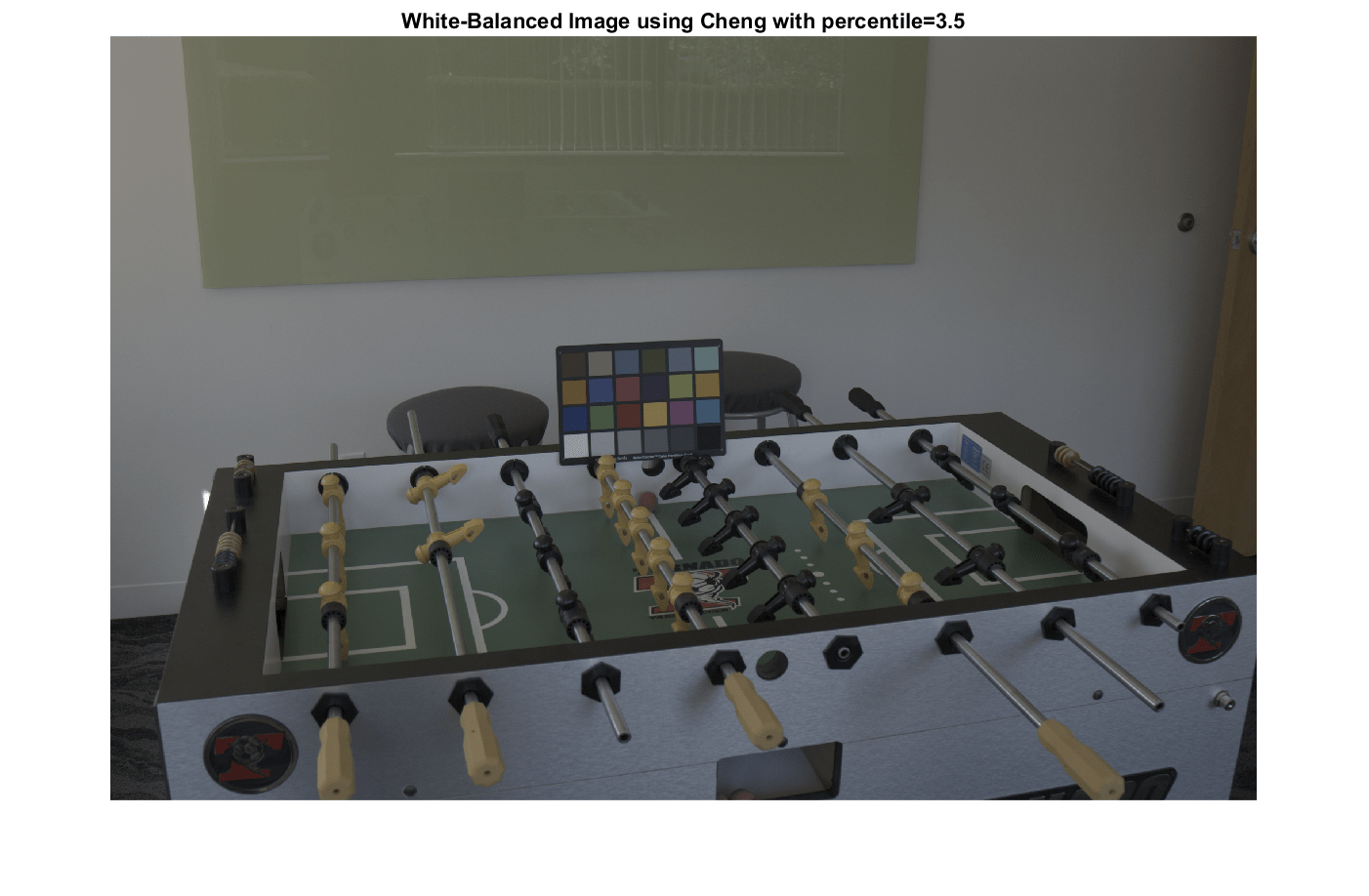Find the best parameter for each algorithm.

```[~,idx_best] = min(err); best_param_wp = param_range(idx_best(1)); best_param_gw = param_range(idx_best(2)); best_param_ch = param_range(idx_best(3)); fprintf('The best parameter for White Patch is %1.2f with angular error %1.2f degrees\n', ... best_param_wp, err(idx_best(1),1));```
```The best parameter for White Patch is 0.25 with angular error 3.33 degrees ```
```fprintf('The best parameter for Gray World is %1.2f with angular error %1.2f degrees\n', ... best_param_gw, err(idx_best(2),2));```
```The best parameter for Gray World is 0.00 with angular error 5.06 degrees ```
```fprintf('The best parameter for Cheng is %1.2f with angular error %1.2f degrees\n', ... best_param_ch, err(idx_best(3),3));```
```The best parameter for Cheng is 0.50 with angular error 1.72 degrees ```

Compute and display the best estimated illuminant for each algorithm in RGB space.

```best_illum_wp = illumwhite(A, best_param_wp, 'Mask', ~mask_chart); best_illum_gw = illumgray(A, best_param_gw, 'Mask', ~mask_chart); best_illum_ch = illumpca(A, best_param_ch, 'Mask', ~mask_chart); plot3([0 1],[0 1],[0,1],'LineStyle',':','Color','k') hold on plot3(... [0 illuminant_groundtruth(1)/norm(illuminant_groundtruth)], ... % Red [0 illuminant_groundtruth(2)/norm(illuminant_groundtruth)], ... % Green [0 illuminant_groundtruth(3)/norm(illuminant_groundtruth)], ... % Blue 'Marker','.', 'MarkerSize',10) plot3( ... [0 best_illum_wp(1)/norm(best_illum_wp)], ... % Red [0 best_illum_wp(2)/norm(best_illum_wp)], ... % Green [0 best_illum_wp(3)/norm(best_illum_wp)], ... % Blue 'Marker','.', 'MarkerSize',10) plot3( ... [0 best_illum_gw(1)/norm(best_illum_gw)], ... % Red [0 best_illum_gw(2)/norm(best_illum_gw)], ... % Green [0 best_illum_gw(3)/norm(best_illum_gw)], ... % Blue 'Marker','.', 'MarkerSize',10) plot3( ... [0 best_illum_ch(1)/norm(best_illum_ch)], ... % Red [0 best_illum_ch(2)/norm(best_illum_ch)], ... % Green [0 best_illum_ch(3)/norm(best_illum_ch)], ... % Blue 'Marker','.', 'MarkerSize',10) xlabel('R') ylabel('G') zlabel('B') title('Best illuminants in RGB space') xlim([0 1]) ylim([0 1]) zlim([0 1]) view(28, 36) legend('achromatic line', 'ground truth', 'White Patch', 'Gray World', 'Cheng') grid on axis equal```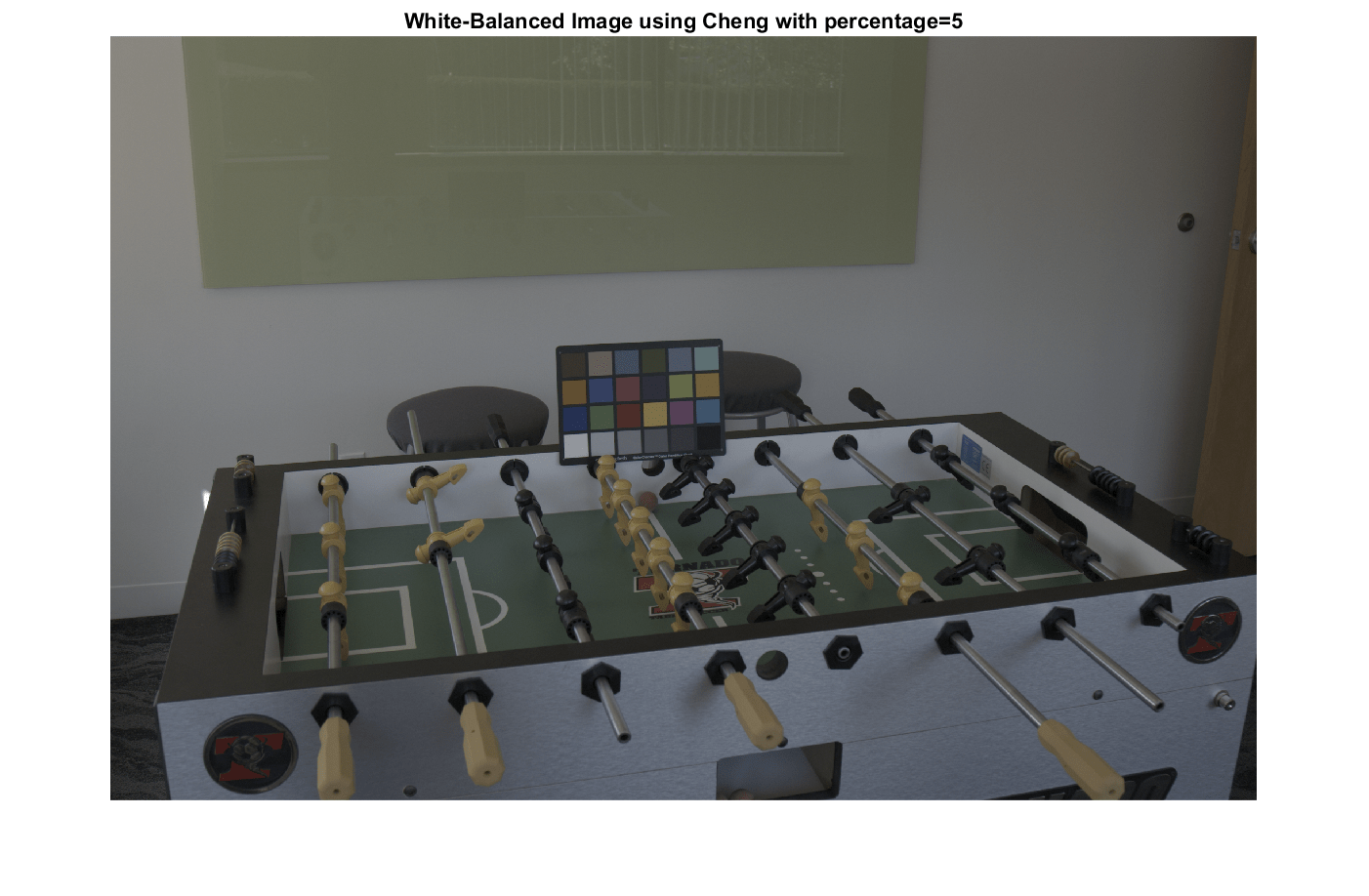Display the white balanced images for each method using the best illuminant side by side.

```B_wp_best = chromadapt(A, best_illum_wp, 'ColorSpace', 'linear-rgb'); B_wp_best_sRGB = lin2rgb(B_wp_best); B_gw_best = chromadapt(A, best_illum_gw, 'ColorSpace', 'linear-rgb'); B_gw_best_sRGB = lin2rgb(B_gw_best); B_ch_best = chromadapt(A, best_illum_ch, 'ColorSpace', 'linear-rgb'); B_ch_best_sRGB = lin2rgb(B_ch_best); M = zeros(size(A,1), 3*size(A,2), size(A,3), 'like', A); M(:,1:size(A,2),:) = B_wp_best_sRGB; M(:,size(A,2)+1:2*size(A,2),:) = B_gw_best_sRGB; M(:,2*size(A,2)+1:end,:) = B_ch_best_sRGB; figure imshow(M) title('Montage of the best white balanced images: White Point, Gray World, Cheng')```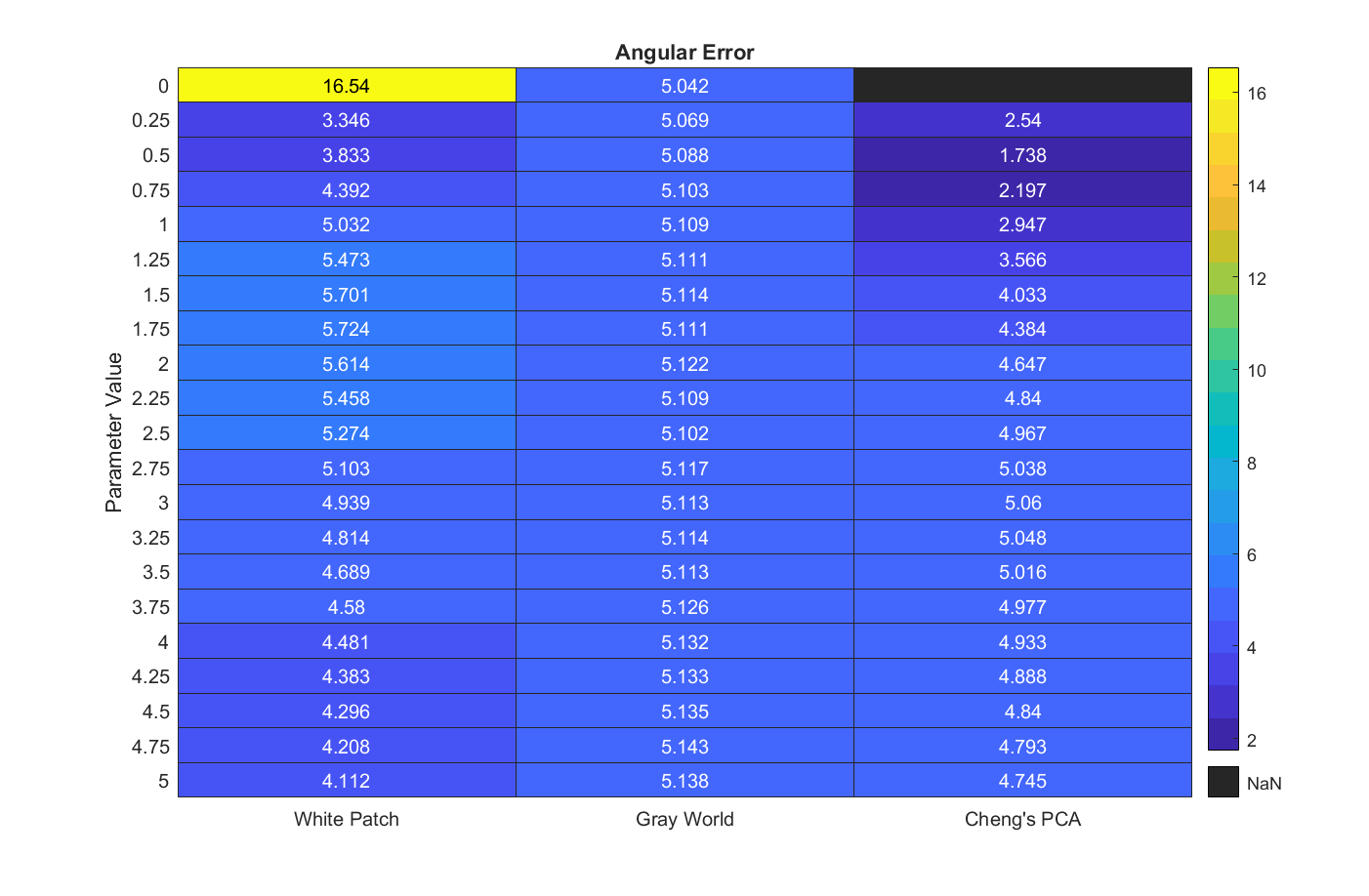### Conclusion

This quick shootout between two classic illuminant estimation methods and a more recent one shows that Cheng's method, using the top and bottom 0.75% darkest and brightest pixels, wins for that particular image. However, this result should be taken with a grain of salt.

First, the ground truth illuminant was measured using a ColorChecker Chart and is sensitive to shot and sensor noise. The ground truth illuminant of a scene can be better estimated using a spectrophotometer.

Second, we estimated the ground truth illuminant as the mean color of the neutral patches. It is also common to use the median instead of the mean. Doing so could shift the ground truth by a significant amount. For example, for the image in this study, using the same pixels, the median color and the mean color of the neutral patches are 0.5 degrees apart, which in some cases can be more than the angular error of the illuminants estimated by different methods.

Third, a full comparison of illuminant estimation methods should use a variety of images taken under different conditions. One method might work better than the others for a particular image, but might perform poorly over the entire data set.

### References

 Ebner, Marc. White Patch Retinex, Color Constancy. John Wiley & Sons, 2007. ISBN 978-0-470-05829-9.

 Ebner, Marc. The Gray World Assumption, Color Constancy. John Wiley & Sons, 2007. ISBN 978-0-470-05829-9.

 Cheng, Dongliang, Dilip K. Prasad, and Michael S. Brown. "Illuminant estimation for color constancy: why spatial-domain methods work and the role of the color distribution." JOSA A 31.5 (2014): 1049-1058.

 Van De Weijer, Joost, Theo Gevers, and Arjan Gijsenij. "Edge-based color constancy." IEEE Transactions on image processing 16.9 (2007): 2207-2214.